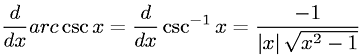Equations > Calculus > Differentiation/Differentals > Derivative of Inverse Cosecant (Arccosecant)

### Derivative of Inverse Cosecant (Arccosecant)Latex Code:

MathML Code:

 $\fracd\mathrm{dx}\mathrm{arc}\mathrm{csc}x=\fracd\mathrm{dx}{\mathrm{csc}}^{-1}x=\frac-1|x|\sqrt{{x}^{2}-1}$

MathType 5.0: